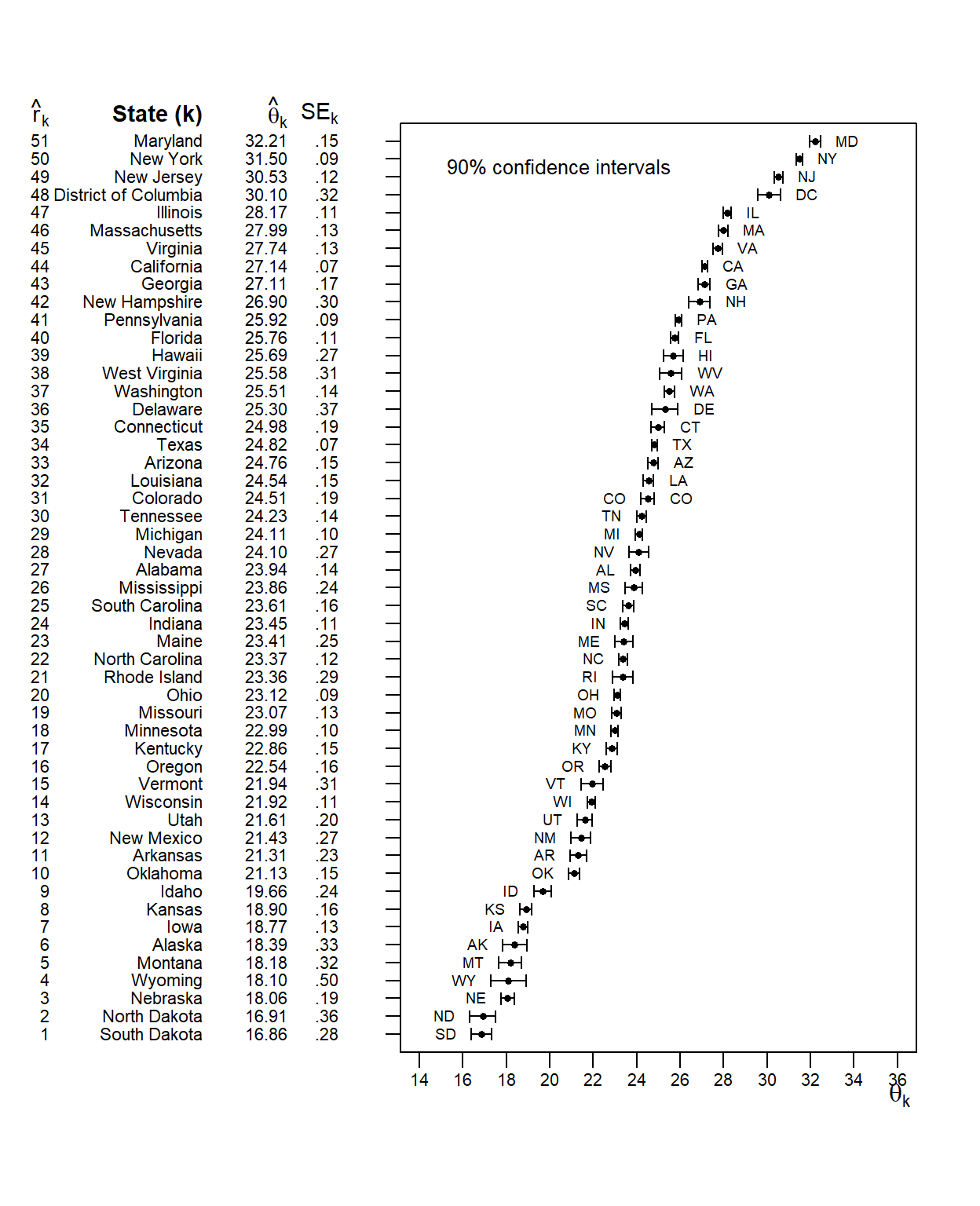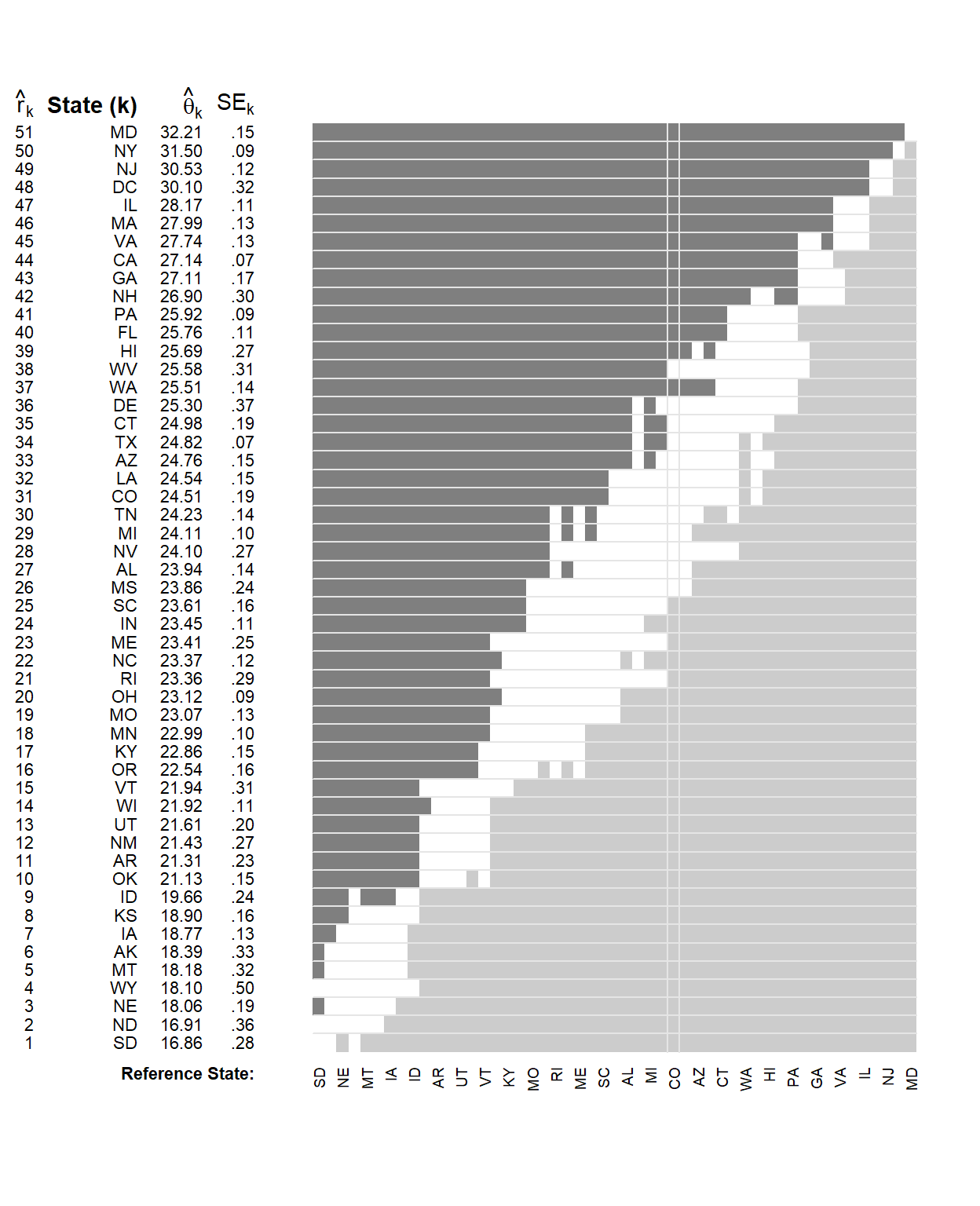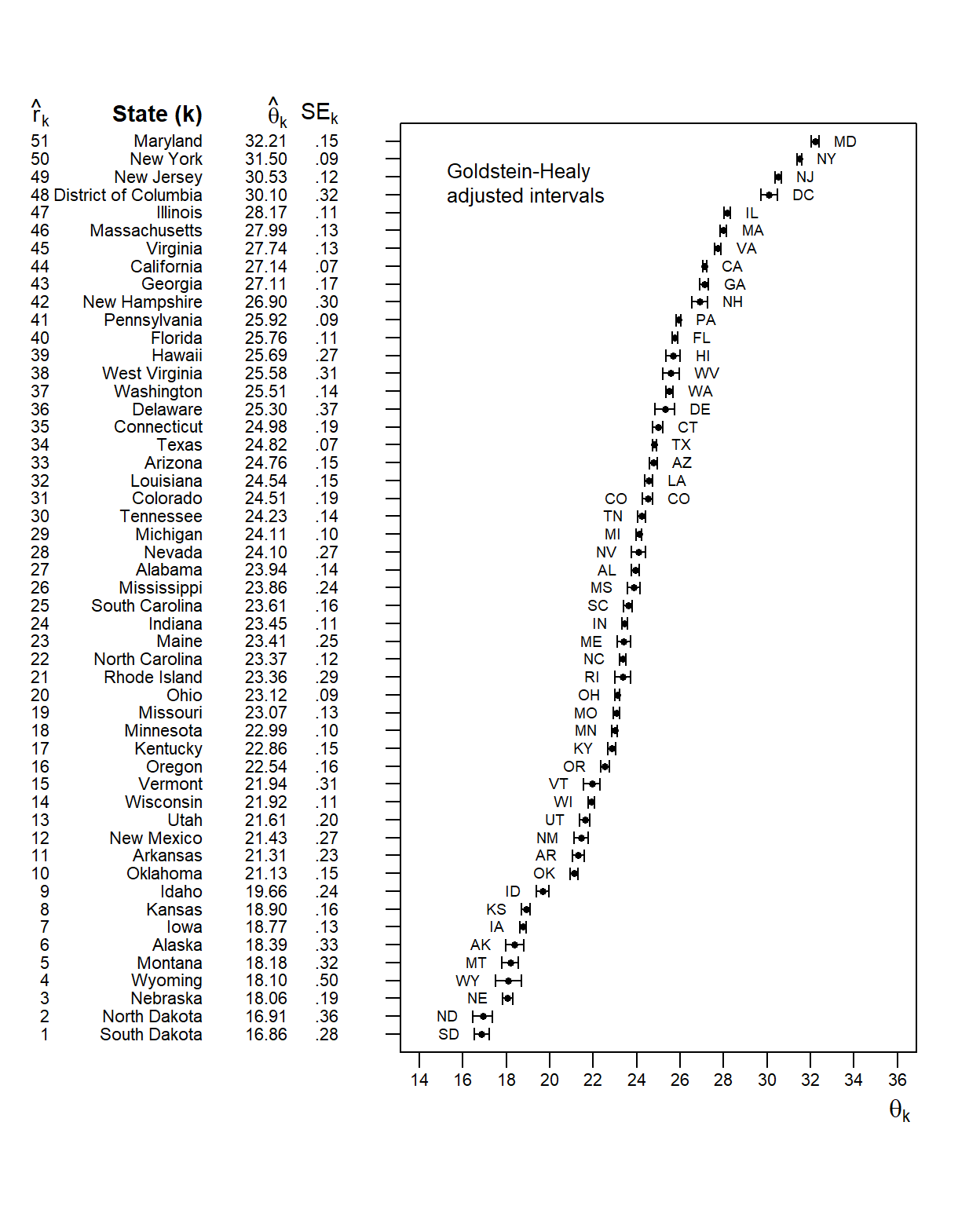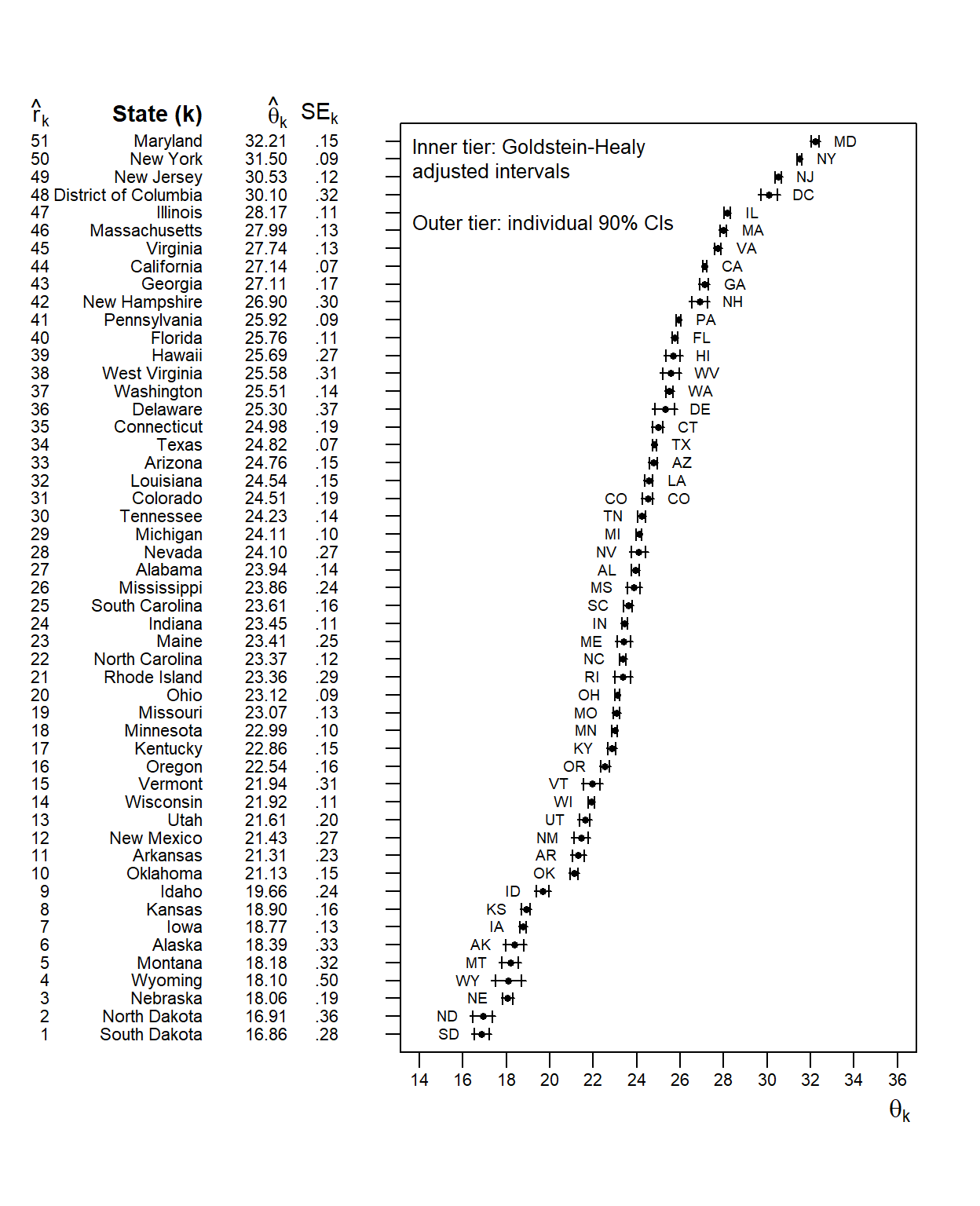This vignette introduces the RankingProject package, which accompanies the articles “A Primer on Visualizations for Comparing Populations, Including the Issue of Overlapping Confidence Intervals” (Wright, Klein, and Wieczorek, 2019, The American Statistician) and “A Joint Confidence Region for an Overall Ranking of Populations” (Klein, Wright, and Wieczorek, 2020, Journal of the Royal Statistical Society: Series C). For instructions on exactly replicating the article’s main figures, please see the Primer and Joint vignettes:
vignette("primer", package = "RankingProject") and vignette("joint", package = "RankingProject")

The package provides functions for plotting ranked tables of data side-by-side with their plots. The available visualizations include shaded columns plots, adjusted confidence intervals, and related plots intended for making correct inferences about one-to-many or many-to-many comparisons.

## Data preparation and a simple figure

First, we load the package and the TravelTime2011 dataset used in the paper. This dataset contains 51 rows (estimates for each of the 50 US states and Washington, D.C.) and 7 columns. The variables Estimate.2dec and SE.2dec are estimates of the mean and its standard error for the mean travel time (in minutes) to work of workers 16 years and over who did not work at home, from the 2011 American Community Survey (ACS).

We also create string versions of our estimates and their standard errors, for printing out tables with a consistent number of digits.

library(RankingProject)
data(TravelTime2011)
USdata  <- TravelTime2011
head(USdata)
##   Rank        State Estimate.2dec SE.2dec Abbreviation  Region FIPS
## 1    1 South Dakota         16.86    0.28           SD MIDWEST   46
## 2    2 North Dakota         16.91    0.36           ND MIDWEST   38
## 3    3     Nebraska         18.06    0.19           NE MIDWEST   31
## 4    4      Wyoming         18.10    0.50           WY    WEST   56
## 5    5      Montana         18.18    0.32           MT    WEST   30
## 6    6       Alaska         18.39    0.33           AK PACIFIC    2
# Format estimates and SEs into strings with 2 digits past the decimal
USdata$Estimate.Print = formatC(USdata$Estimate.2dec,
format = 'f', digits = 2)
# For SEs, also drop the leading 0
USdata$SE.Print = substring(formatC(USdata$SE.2dec,
format = 'f', digits = 2),
first = 2)

Next, we set up several list-type objects to contain parameters needed for the tables and plots. We will use Colorado (CO) as the reference area. In particular, to plot individual confidence intervals, we set plotType="individual" inside plotParList.

# Set Colorado as the reference area
refAbbr <- "CO"
refRow  <- which(USdata$Abbreviation==refAbbr) # Set up parameter lists for table function and plot function tableParList <- with(USdata, list(ranks = Rank, names = State, est = Estimate.Print, se = SE.Print, placeType = "State")) plotParList <- with(USdata, list(est = Estimate.2dec, se = SE.2dec, names = Abbreviation, refName = refAbbr, confLevel = .90, cex = 0.6, plotType = "individual")) Finally, we create a simple plot of individual 90% confidence intervals (CIs), alongside the ranking table: # Individual CIs RankPlotWithTable(tableParList = tableParList, plotParList = plotParList)However, such individual 90% confidence intervals are not explicitly designed for making comparisons. As noted in the article, when reading individual CIs it is tempting to conclude that states with overlapping intervals are not significantly different at the $$\alpha=0.10$$ significance level, but this is not necessarily true. The RankingProject package includes several other plot types that are specifically designed for making inferences from a plot appropriately. Notes: if we set plotParList$refName=NULL for the individual CIs plot above, the state abbreviations will flip sides around the median state instead of around the reference state. And if we wish, we can set plotParList$legendText <- NA to remove the default legend. ## Shaded columns plot First, we can use plotParList$plotType="columns" to create a grid or “shaded columns plot” that simply shows which states are significantly different from one another. We start with significance level $$\alpha=0.10$$ and apply a “demi-Bonferroni” correction for 50 comparisons between one reference state and all other states. Then we perform these 50 one-to-many tests within each column of the plot, testing the reference state (labeled at the bottom of the column) against each other state at significance level $$\alpha/50 = 0.002$$.

(The intended audience behind this demi-Bonferroni correction is a reader who already has one reference state in mind, such as his or her home state, and does not care about comparisons that exclude this particular state. For more general all-to-all comparisons, the 50-way demi-Bonferroni correction could be replaced with a $${51 \choose 2}$$-way full-Bonferroni correction.)

# Shaded Columns plot
plotParList$plotType <- "columns" # Specify where to position the "Reference State:" text, # and adjust column widths from their defaults tableParList <- c(tableParList, list(columnsPlotRefLine = .7, col2 = .55, col3 = .8)) # Use abbreviations instead of full names in the table, to save space tableParList$names <- USdata$Abbreviation # Use default plotting character size plotParList$cex <- NULL

# Actually draw the figure
RankPlotWithTable(tableParList = tableParList, plotParList = plotParList,
tableWidthProp = 2/7)# Reset defaults for future plots
tableParList[c("columnsPlotRefLine", "col2", "col3")] <- NULL
# Use full state names in the table for future plots
tableParList$names <- USdata$State

The vertical lines highlighting Colorado are optional and could be removed by setting plotParList$refName=NULL. ## Individual, Goldstein-Healy adjusted, and Bonferroni-corrected CIs We can improve the first plot (of individual CIs) by performing a Goldstein-Healy adjustment, as summarized in the article and originally proposed by Goldstein and Healy (1995). Using this adjustment, instead of plotting actual 90% CIs, we plot CIs at a different confidence level which is chosen (based on the standard errors of all the estimates) to achieve an “average significance level” of 0.10. This lets us actually perform inferences by checking for overlap of intervals. If two intervals do (do not) overlap, they are not (are) significantly different at this “average significance level.” Usual CIs do not have this property. # Set smaller plot text and lower x-axis label for future plots plotParList$cex <- 0.6
plotParList$thetaLine <- 1.5 # Goldstein-Healy adjusted CIs plotParList$plotType <- "individual"
plotParList$GH <- TRUE RankPlotWithTable(tableParList = tableParList, plotParList = plotParList)We could show both the usual CIs and the Goldstein-Healy-adjusted versions on a single plot, by using two-tiered error bars. Here, the inner tier (between the cross bars) shows the Goldstein-Healy adjustment, and the outer tier (all the way to the ends of the intervals) shows the individual 90% CIs. When we set plotParList$tiers=2 the plot function will always show individual confidence intervals on one tier, and Goldstein-Healy and/or Bonferroni adjustments on another tier. The legend will automatically show which tier is which (since adjusted intervals could either all be shorter or all be longer than the un-adjusted intervals, depending on which adjustments are made).

# Double-tiered GH plot:
# inner tiers are GH CIs,
# outer tiers are usual 90% CIs
plotParList$tiers <- 2 # Legend auto-positioning is poor with line breaks in legend text; # we can improve it by controlling (X,Y) manually plotParList$legendX <- 12
plotParList$legendY <- 53 RankPlotWithTable(tableParList = tableParList, plotParList = plotParList)However, the Goldstein-Healy adjustment allows for visual inspection of overlap, but does not correct for multiple comparisons. If we have one reference state in mind, we may wish to use a demi-Bonferroni correction for comparing one state against the other 50: # Double-tiered GH + Bonferroni plot: # inner tiers are usual 90% CIs, # outer tiers are 50-way demi-Bonferroni-corrected GH CIs plotParList$multcomp.scope <- "demi"
RankPlotWithTable(tableParList = tableParList, plotParList = plotParList)